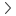# Swap Calculator

Traders' toolsSwap calculator

Synthetic

Financial

0

### How to calculate swap charges

For synthetic, the swap charge is calculated on an annual basis for long and short positions based on this formula:

Swap charge = volume × contract size × asset price × (swap rate ÷ 100) ÷ 360

This gives you the swap charge in USD.

### Example calculation

Let’s say you want to keep 0.01 lots of Volatility 75 Index with an asset price of 400,000 USD and swap rate of -7.5 open for one night.1. The contract size is one standard lot of Volatility 75 Index = 1
2. If the swap rate is positive, your account will be credited with the swap amount. If it is negative, your account will be debited.

So you will require a swap charge of 0.83 USD to keep the position open for one night.